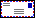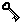Name: Ettore Minguzzi
Position: Associate Professor MAT/07-Mathematical Physics (with 2012 Italian full professor qualification)  at

Università degli Studi di Firenze,
Facoltà di Ingegneria,
Dipartimento di Matematica e Informatica "U. Dini",
Via S. Marta 3,
I-50139 Firenze, Italia
Tel. +39 055 2758 925
Fax. +39 055 471787My pgp key

Research interests: General relativity, global Lorentzian and Lorentz-Finsler geometry, topological preordered spaces.

NEWS:  International Meeting on Non-regular spacetime geometry

Scientific results:
(for references email me or check my publication list)

Mathematical Relativity and topological ordered spaces:

• Definition and development of affine sphere spacetimes
• Positive solution of an old conjecture concerning the smoothness of compact Cauchy horizons under positive energy conditions. Consequent results on the fact that under reasonable conditions Lorentzian cobordisms are trivial (no topology change), and closed timelike curves cannot form (no time machines). Proof that Cauchy horizons with compact components cannot form from reasonable initial data (a case of strong cosmic censorship conjecture).
• Strengthening of Hawking's area theorem for horizons.
• Proof that convex normal globally hyperbolic neighborhoods exist.
• Extension of singularity theorems to the Finsler case.
• Proof that causality theory can be generalized to the Finslerian case and/or to the Lipschitz connection  case, extending proofs word by word (whenever curvature is not involved).
• Proof of the reverse Cauchy-Schwarz and reverse triangle inequality for Lorentz-Finsler geometry with metric defined on the slit tangent bundle or on conic subbundles.
• Proof that the Lorentz-Finsler theory for metrics defined on the slit tangent bundle predicts two lightlike cones as desired provided the spacetime dimension is larger than two.
• Proof that locally Lipschitz and anti-Lipschitz time functions can be approximated by smooth time functions with timelike gradient. Also Hawking's time function can be so approximated.
• Proof of the stability of global hyperbolicity.
• Proof that any topological preordered space endowed with a closed order determined by local data (e.g. cone structure) in which the topology is sufficiently good is quasi-uniformizable, namely topology and order can be recovered from the sets of isotone (non-decreasing) functions. Thus these spaces admit a Nachbin compactification.
• Proof that stably causal spacetimes are quasi-uniformizable (the causal order and the topology follow from the set of time functions) and that globally hyperbolic spacetimes are even quasi-pseudo-metrizable. Thus stably casusal spacetimes can be compactified.
• Proof that every closed preordered space endowed with a locally compact sigma-compact topology is normally preordered. Under second countability it admits a countable utility representation.
• Realization that there is a nice relationship between economics results on utility theory and relativistic results on time functions.
• Proof of a novel type of singularity theorem which states that under standard genericity and positive energy conditions either the spacetime admits a time function or it has pathologies in the form of chronology violation or lightlike geodesic singularities.
• Positive solution of Low's conjecture on the equivalence between Sorkin and Woolgar's K-causality and Hawking's stable causality.
• Clarification of the causal ladder, e.g. through the introduction of the non-imprisonment conditions.
• Development of limit curve theorems without casuality conditions.
• Proposal that the Big Bang is a lightlike boundary of a chronology violating set. This model nicely solves the homogenity/isotropy and entropy problems of cosmology.
• Extension of the Avez-Seifert geodesic connectedness theorem to the Lorentz force case.
• Classification of  the conjugacy classes of the inhomogeneous Lorentz group and proof of  a relativistic Chasles theorem.

Other
• Proof that the exponential map for Lipschitz connections or sprays is strongly differentiable at the origin and provides a local lipeomorphism.
• Introduction of a third order equation alternative to the Lagrange equation and introduction of the Power Lagrangian.
• Clarification of simultaneity issues using the concept of round-trip time and proposal of alternative synchronization schemes.